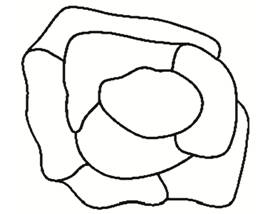# Map Coloring, a fictional map is given showing the states of a country. Represent the map by a graph and find a coloring of the graph, using the minimum number of colors possible, such that no two connected vertices are the same color. Then color the map according to the graph coloring that you found.### Mathematical Excursions (MindTap C...

4th Edition
Richard N. Aufmann + 3 others
Publisher: Cengage Learning
ISBN: 9781305965584

#### Solutions

Chapter
Section### Mathematical Excursions (MindTap C...

4th Edition
Richard N. Aufmann + 3 others
Publisher: Cengage Learning
ISBN: 9781305965584
Chapter 5, Problem 29RE
Textbook Problem
1 views

## Map Coloring, a fictional map is given showing the states of a country. Represent the map by a graph and find a coloring of the graph, using the minimum number of colors possible, such that no two connected vertices are the same color. Then color the map according to the graph coloring that you found.To determine

To represent:

The map by graph and find the color with minimum possibilities

### Explanation of Solution

Given:

Calculation:

Assume the below given diagram as map with countries of a continent

Draw vertex for each country

And now connect the vertices and edges and see to that it is connected to nearby countries

### Still sussing out bartleby?

Check out a sample textbook solution.

See a sample solution

#### The Solution to Your Study Problems

Bartleby provides explanations to thousands of textbook problems written by our experts, many with advanced degrees!

Get Started

Find more solutions based on key concepts
In Exercises 85-88, determine whether the statement is true or false. If it is true, explain why it is true. If...

Applied Calculus for the Managerial, Life, and Social Sciences: A Brief Approach

Show that if an 0 and limn nan 0, then an is divergent.

Single Variable Calculus: Early Transcendentals

What information should be included in the abstract of an APA-style research report?

Research Methods for the Behavioral Sciences (MindTap Course List)

Find for y defined implicity by .

Study Guide for Stewart's Multivariable Calculus, 8th

Which is true about the series ? diverges converges absolutely converges conditionally converges, but not absol...

Study Guide for Stewart's Single Variable Calculus: Early Transcendentals, 8th